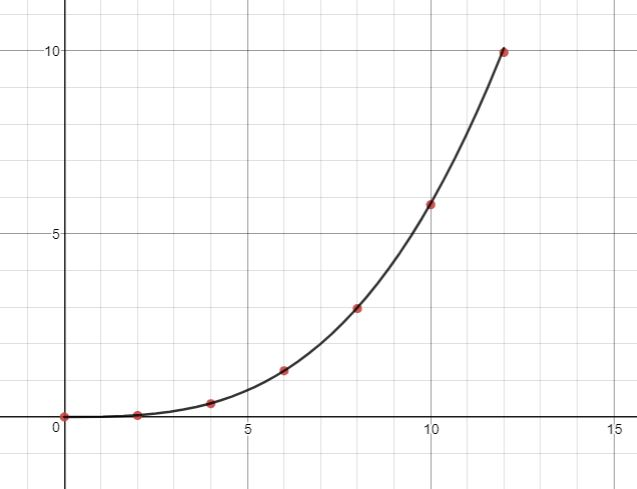Why is the power of a filament lamp directly proportional to the cube of its voltage?

I was doing a textbook question on how the power of a bulb varies with the potential difference across it. I plotted this graph:(V is on the x axis and P is on the y axis.)

I was then told that this graph obeys the relationship $$P=kV^3$$ (note that for ohmic conductors the relationship is $$P=kV^2$$, where $$k=\frac1 R$$), and I was then told to explain this relationship. I'm not terribly sure where to start; I know that increasing the voltage increases the temperature of the filament and therefore the resistance, but doesn't that mean the current decreases, therefore decreasing the power of the bulb?

For those of you in the British education system, I'm just starting my A level in Physics (which means I'm 17 years old for everyone else), so a decently non-technical answer would be appreciated...

• Something to think about: 1) Is this an ohmic material? 2) How is the resistance of this material mathematically related to the voltage across the material? 3) Is this a material that you would commonly expect to find? Oct 25 '18 at 14:23
• Who told you this (third power)) I recently had a student plot the same graph and it was rather proportional to the 1.5th power. Matching your thougts. Oct 25 '18 at 14:25
• The book I was using has sections to test practical skills and this question was in there. Maybe they stated this with some given assumptions, but for some reason it seems to make at least some sense... Oct 25 '18 at 14:30
• Yeah that $kV^3$ dependency you might see in a thermistor under the assumption that conductance can be linearized so $R=R_0/(1 + \epsilon~V)$ and then $P=V^2/R = V^2/R_0 + \epsilon~V^3/R_0$... if your conductance is not increasing linearly with $V$ then this behavior would seem inexplicable. Oct 25 '18 at 14:34
• I think you have to include (a picture of) the exact question and the source of the $~^3$ claim. Oct 25 '18 at 16:23

Think of it the following way. You were right in writing the relationship between power, voltage and resistance:

$$P=\frac{V^2}{R}.$$

But this equation was said to not fit the data, and instead

$$P=kV^3.$$

Comparing these two equations, we arrive at the relationship

$$R=\frac{1}{kV}.$$

This is the thing we need to explain. As you've hinted, it's related to the temperature dependence of the material.

Consider the filament to be a black body of area $$A$$ and at temperature $$T$$. The electrical power going through it should be irradiated out through black body radiation. We then say

$$P=\sigma AT^4,$$

Where $$\sigma$$ is the Stefan-Boltzmann constant. Comparing this to the expression given for the power

$$kV^3=\sigma AT^4$$

$$V=\left(\frac{\sigma A}{k}\right)^{1/3}T^{4/3}.$$

Substituting this into our expression for the resistance we get

$$R=\left(\frac{1}{k^2\sigma A}\right)^{1/3} T^{-4/3}.$$

This is quite odd, to be honest. It implies the resistance decreases with temperature, which is quite the opposite of what we have with conductors usually. Are you sure the power goes as $$V^3$$?

• The strange thing was that the table I used to plot the graph seemed to suggest the increase in current was rising per unit increase in voltage... which, like you say, is quite strange (e.g., the currents at 8.00V, 10.00V and 12.00V were 0.37A, 0.58A and 0.83A, respectively). Maybe the book was making this relationship up? I'll wait for more answers. Oct 25 '18 at 15:05
• @Cr0xx Maybe the curve above is actually a voltage-vs-current curve. The axes aren't labeled anyway. Oct 25 '18 at 15:54
• For this answer, are you sure the relation $P=\frac{V^2}{R}$ holds for non-Ohmic materials? After all, it's derived from the more fundamental $P=VI$ using Ohm's Law. Oct 25 '18 at 15:55
• @probably_someone $P=VI$ does not depend on Ohm's Law. It is just a statement that says the power is the number of charges per unit time (current) losing some energy per unit charge (voltage). It is simply work per unit time. Resistance, on the other hand, is defined as $V=RI$, even though it can depend on both $V$ and $I$ in weird ways. $P=V^2/I$ still holds. Oct 25 '18 at 15:59
• The first two equations that you wrote down imply that R decreases with temperature (or increasing voltage). For P to increase at a faster rate than $V^2$, R has to be decreasing with increasing voltage (or temperature). So the filament material would not be a standard metal filament but should be thought of as some sort of semiconducting filament which was a negative temperature coefficient of resistivity. Don't even know if semiconducting filaments are workable in reality or if they would suffer from a thermal runaway problem but that's what a $P=kV^3$ behavior corresponds to.
– user93237
Oct 25 '18 at 18:06

A better way to look at this problem is to see that the power used by the tungsten filament is actually proportional to the voltage to the 1.54 power.

The graph you show, has the power proportional to the voltage to the 3.2 power. This is unphysical and typical of physics textbook writers who post hypothetical problems.

The source for this equation and other lighting questions can be found in The "Lighting Handbook: Reference and Application" (8th ed.) from the IES (Illuminating Engineering Society) p.186

• Welcome to Physics! This should probably be edited into your previous answer, rather than posted as a new answer. Please use the "Edit" link below your first answer to do so; I would then recommend that you delete this one. Jan 15 '21 at 21:45